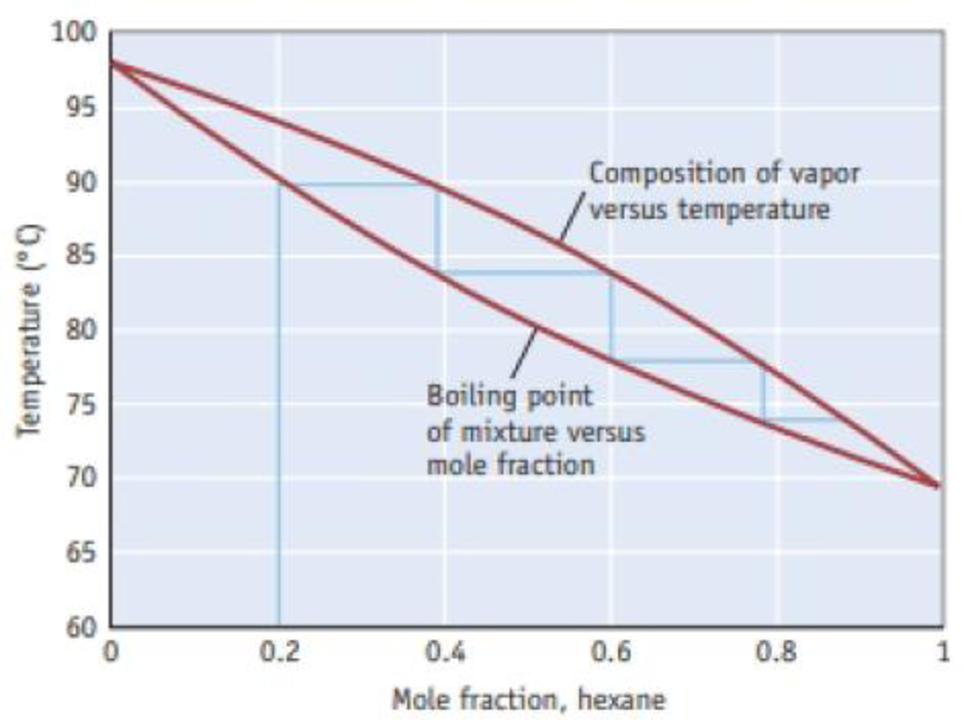# The blue line on the diagram illustrates the effect of using fractional distillation to separate a mixture of hexane (C 6 H 14 ) and heptane (C 7 H 16 ). If one starts a fractional distillation with a 0.20 mole fraction of hexane, what is the approximate mole fraction of hexane in the vapor phase after two evaporation condensation cycles? Figure B A temperature–composition diagram for hexane (C 6 H 14 )-heptane (C 7 H 16 ) mixtures.### Chemistry & Chemical Reactivity

9th Edition
John C. Kotz + 3 others
Publisher: Cengage Learning
ISBN: 9781133949640

#### Solutions

Chapter
Section### Chemistry & Chemical Reactivity

9th Edition
John C. Kotz + 3 others
Publisher: Cengage Learning
ISBN: 9781133949640
Chapter 13.5, Problem 1Q
Textbook Problem
200 views

## The blue line on the diagram illustrates the effect of using fractional distillation to separate a mixture of hexane (C6H14) and heptane (C7H16). If one starts a fractional distillation with a 0.20 mole fraction of hexane, what is the approximate mole fraction of hexane in the vapor phase after two evaporation condensation cycles?Figure B A temperature–composition diagram for hexane (C6H14)-heptane (C7H16) mixtures.

Interpretation Introduction

Interpretation: Approximate mole fraction of hexane in the vapor phase after two evaporation –condensation cycles has to be determined from the given diagram.

Concept introduction:

• Mole fraction is one of the concentrations expressing term which is equal to the number of moles of a component divided by the total number of a solution. The mole fraction of all components of a solution, when added together will equal to 1

The mole fraction of component a can be determined by using the equation,

Molefractionofa(Xa)=MoleofaTotalmoles

• Fractional distillation it is a separation process in which the volatile components of a mixture are split from one another by heating the mixture in a column and collecting and condensing the vapors drawn from different levels of the column.
• Boiling point is the temperature at which the vapor pressure of the substance comes equal to the atmospheric pressure.
• In evaporation matter changes from a liquid to a gas and in condensation matter changes from a gas to a liquid.

### Explanation of Solution

Temperature – composition diagram for hexane (C6H14) – heptane (C7H16 ) mixtures.

Here the lower curved line indicates the boiling point of the mixture as a function of mole fraction of the more volatile component, hexane. The upper curve indicates the mole fraction of hexane in the vapor phase above the boiling solution

### Still sussing out bartleby?

Check out a sample textbook solution.

See a sample solution

#### The Solution to Your Study Problems

Bartleby provides explanations to thousands of textbook problems written by our experts, many with advanced degrees!

Get Started

Find more solutions based on key concepts
Which of the following is a physical consequence of fasting? a. loss of lean body tissues b. lasting weight los...

Nutrition: Concepts and Controversies - Standalone book (MindTap Course List)

Two single-turn circular loops of wire have radii R and r, with R r. The loops lie in the same plane and are c...

Physics for Scientists and Engineers, Technology Update (No access codes included)

What is the difference between sea islands and barrier islands?

Oceanography: An Invitation To Marine Science, Loose-leaf Versin